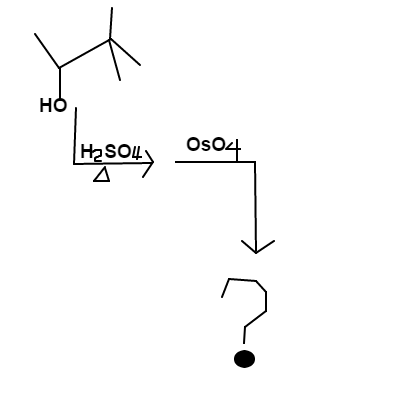# Alcoholic Coordination

Chemistry Level 2$\text{t-butyl ethanol}$ is mixed into a solution of heated $\text{sulfuric acid}$ and the major product is mixed with $\text{osmium tetroxide}$. The final product will contain one or more alcohol substituents. Your answer should be the sum of the carbon positions ($C_{OH}$) an alcohol is attached. For example, if $C_1$, $C_3$, and $C_9$ all have alcohol groups, then your answer would be $1+3+9 = \boxed{13}$.  Use IUPAC numbering protocol when labeling the carbon positions in your answer.

David's Organic Chemistry Set

David's Physical Chemistry Set

×

Problem Loading...

Note Loading...

Set Loading...